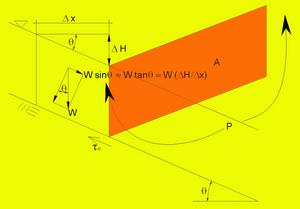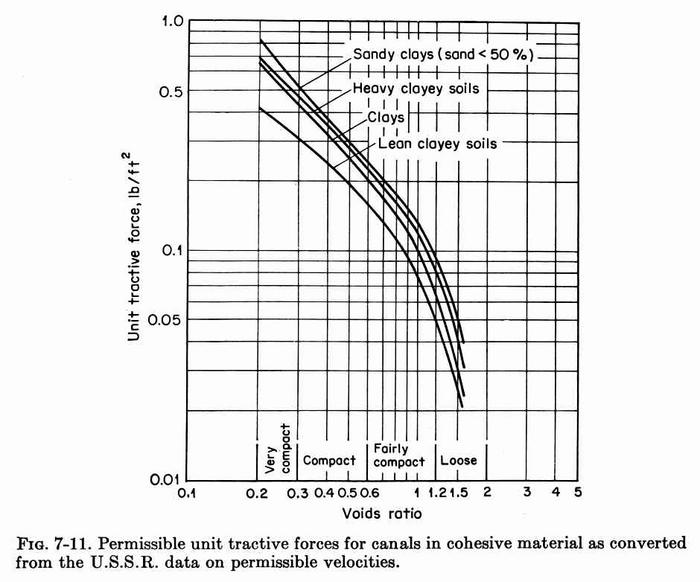CIVE 530 - OPEN-CHANNEL HYDRAULICS LECTURE 7A: DESIGN OF CHANNELS FOR UNIFORM FLOW II

 7.11 THE TRACTIVE FORCETractive Force Sketch

• Assume a control volume of area A, wetted perimeter P, and distance (channel length) Δx, with channel head drop ΔH:

• Assume a channel slope So, equal to:

 So = ΔH / Δx = tan θ

• The total weight of the water in the control volume (gravitational force) is:

 W = γ A Δx

• The tractive force is:

 Ft = W sinθ ≅ W tan θ = γ A Δx So

• The resisting force is:

 Fr = τo P Δx

• Equating tractive and resisting force leads to:

 τo = γ (A/P) So = γ R So

• For hydraulically wide channels (R ≅ y):

 τo = γ y So

• The distribution of the tractive stress varies along the wetted perimeter.

• At the channel bottom, the maximum tractive force is the full value:  γySo.

• At the channel sides, the maximum tractive force is only:  0.78 γySo.Fig. 7-6 (Chow)Fig. 7-7 (Chow)

 7.12 TRACTIVE FORCE RATIO

• Two forces are acting on a soil particle of submerged weight Ws resting on the side of a channel, with tractive stress τs and angle of inclination of the slope φ:

1. The tractive force: aτs    (a= effective area of the particle).

2. The gravity force component: Wssinφ .

• The resultant acting force is:

 Fa = (Ws2sin2φ + a2τs2)1/2

• When this acting force is large enough, the particle will move.

• At equilibrium, the resistance force is equal to the acting force.

• The resistance force is equal to the normal force Wscosφ times the coefficient of friction tanθ, where θ is the angle of friction of the material:

• The resisting force is:

 Fr = Ws cosφ tanθ

• Therefore:

 Ws cosφ tanθ = (Ws2sin2φ + a2τs2)1/2

• Squaring both sides:

 Ws2 cos2φ tan2θ = Ws2sin2φ + a2τs2

 a2τs2 = Ws2 cos2φ tan2θ - Ws2sin2φ

 τs2 = (Ws2/a2) cos2φ tan2θ [1 - (tan2φ/ tan2θ)]

 τs = (Ws/a) cosφ tanθ [1 - (tan2φ/ tan2θ)]1/2

• This is the shear stress on the sides of a channel with side slope angle φ.

• The force balance on a level surface, with φ = 0, is (cosφ = 1; sinφ= 0):

 Ws tanθ = a τL

• Therefore, the shear stress that causes impending motion on a level surface is:

 τL = (Ws/a) tanθ

• The tractive force ratio is defined as:

 K = τs/τL

 K = cosφ [1 - (tan2φ/ tan2θ)]1/2

• This ratio K is only a function of φ and θ.

• Simplifying:

 K = {cos2φ [1 - (tan2φ/ tan2θ)]}1/2

 K = [cos2φ - (cos2φ tan2φ/ tan2θ)]1/2

 K = [cos2φ - (sin2φ/ tan2θ)]1/2

 K = [cos2φ - (sin2φ cos2θ /sin2θ )]1/2

 K = {(1 - sin2φ) - [sin2φ (1 - sin2θ) /sin2θ ]}1/2

 K = {(1 - sin2φ) - [(1 - sin2θ) (sin2φ /sin2θ) ]}1/2

 K = {(1 - sin2φ) - [ (sin2φ /sin2θ) - sin2φ]}1/2

 K = [1 - (sin2φ /sin2θ) ]1/2

• Values of the angle of repose of noncohesive material (sand) are given in Fig. 7-9.

• The diameter is that of a particle for which 25% (by weight) of the material is larger.Fig. 7-9 (Chow)
 7.13 PERMISSIBLE TRACTIVE FORCE

• The permissible tractive force is the maximum shear stress that will not cause erosion of the material forming the channel bed on a level surface.

• The value, obtained from laboratory experiments, is called the critical tractive force.

• USBR practice:

• For coarse noncohesive materials (sand), the permissible tractive force (lbs/sq ft) is equal to 0.4 times the d25 diameter (inches) (See straight line in Fig. 7-10).Fig. 7-10 (Chow)
 For fine noncohesive material (silt), the diameter is the median diameter, or d50. Three designs are recommended: Canals with high content of sediment in the water. Canals with low content of sediment in the water. Canals with clear water ("hungry" water). For cohesive materials, see Fig. 7-11.Fig. 7-11 (Chow)
 The permissible tractive forces mentioned above refer to straight channels. For sinuous channels, the values of permissible tractive force should be lowered to reduce scour. A 10% reduction is recommended for slightly sinuous channels, 25% for moderately sinuous, and 40% for very sinuous. STEPS IN PERMISSIBLE TRACTIVE FORCE METHOD. Assume b/y = 6 and z, with Q, S, and n known. Assume tractive force on sides is critical (as opposed to tractive force on level ground). With b/y and z, enter Fig. 7-7 left to determine Cs in the acting unit tractive force on the sides Ts: Ts = CsγyS With d25 and grain shape, find angle of repose θ from Fig. 7-9. Calculate φ from: tanφ = 1/z Calculate K from: K = [1 - (sin2φ/sin2θ)]1/2 Determine permissible unit tractive force on level ground τL from Fig. 7-10. (If the material differs from sides and bottom, this could be either τLb based on the material on the bottom, or τLs based on the material on the sides). Calculate the permissible unit tractive force on the sides: τs = K τL       [or: τs = K τLs] Set permissible and acting unit tractive forces equal: τs = Ts τs = CsγyS Solve for flow depth y: y = τs/(CsγS) With y and b/y, calculate b = y (b/y) With Q, b, z, S, and n known, design channel to find yn. Test to confirm that: yn ≤ y If not satisfied, assumed b/y is too small. Assume a greater value and return to step 11. If satisfied: y = yn Once b/y is determined by trial and error, enter Fig. 7-7 to determine Cb in the acting unit tractive force on the bottom TL: TL = CbγyS Calculate TL = CbγynS Compare acting unit tractive force TL with permissible unit tractive force on level ground τL calculated in step 7.     [OR: with τLb if different materials] If TL ≤ τL(b), the sides control the design. The design is OK. If TL > τL(b), the bottom controls the design. In this case, make TL = τL(b). Then recalculate yn = τL(b) / (CbγS) With new yn and b/y, recalculate b and confirm yn with ONLINECHANNEL01. Otherwise, assume new b/y until yn calculated with ONLINECHANNEL01 agrees with yn in previous step. PERMISSIBLE TRACTIVE FORCE METHOD: EXAMPLE A (sides and bottom are the same) Given: Q = 600 cfs; b = ?; z = 2; S= 0.001; n = 0.022; sides and bottom: noncohesive material, slightly angular, d25 = 0.7 in. Assume b/y = 6. Assume tractive force on sides is critical (as opposed to tractive force on level ground). With b/y and z, enter Fig. 7-7 left to determine Cs = 0.78. With d25 and grain shape, find angle of repose θ from Fig. 7-9: θ = 34o. Calculate φ from: tanφ = 1/z φ = tan-1 (1/z) = 26.656o. Calculate K from: K = [1 - (sin2φ/sin2θ)]1/2 = 0.6 Determine permissible unit tractive force on level ground τL from Fig. 7-10. τL = 0.4 × d25 (in) = 0.4 × 0.7 = 0.28 psf. Calculate the permissible unit tractive force on the sides: τs = K τL = 0.6 × 0.28 = 0.168 psf. Set permissible and acting unit tractive forces equal: τs = Ts τs = 0.168 = CsγyS = 0.78 × 62.4 × y × 0.001 Solve for flow depth y: y = τs/(CsγS) = 0.168 / (0.78 × 62.4 × 0.001) = 3.45 ft. With y and b/y, calculate b = y (b/y) = 3.45 × 6 = 20.7 ft. Assume b = 21 ft. With Q = 600, b = 21, z = 2, S = 0.001, and n = 0.022 known, use ONLINECHANNEL01 to find yn = 4.356 ft. Test to confirm that: yn = 4.356 > y = 3.45. Normal depth too high! If not satisfied, assumed b/y is too small. Assume a greater value and return to step 11. With b/y = 10, enter Fig. 7-7 to determine Cb = 1.0 Calculate TL = CbγynS = 1.0 × 62.4 × 3.376 × 0.001 = 0.211 psf. Compare acting unit tractive force TL with permissible unit tractive force on level ground τL calculated in step 7. TL = 0.211 < τL = 0.28. Therefore, the sides control the design. The design is OK. PERMISSIBLE TRACTIVE FORCE METHOD: EXAMPLE B (sides and bottom are different) Given: Q = 600 cfs; b = ?; z = 2; S= 0.001; n = 0.022; sides: noncohesive material, slightly angular, d25 = 0.7 in; bottom: noncohesive material, with d50 = 0.8 mm, with high content of fine sediment in the water.

 051019